# Determine the horizontal displacement at A. Take E = 200 GPa. The moment of inertia of...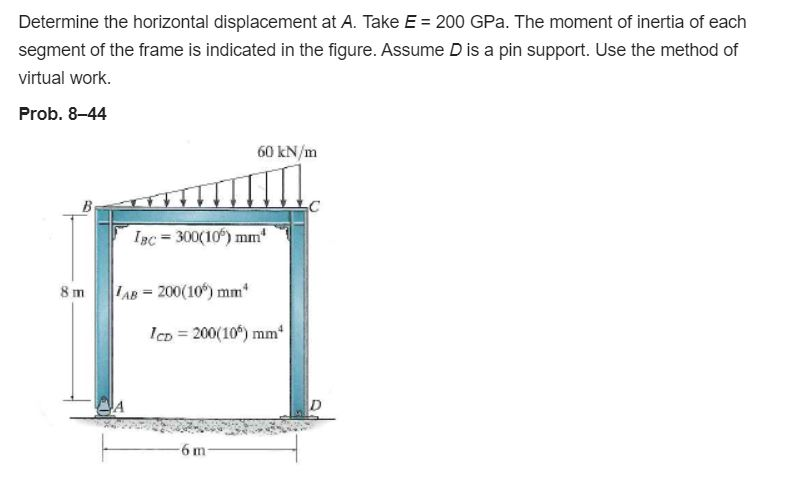Determine the horizontal displacement at A. Take E = 200 GPa. The moment of inertia of each segment of the frame is indicated in the figure. Assume D is a pin support. Use the method of virtual work. Prob. 8-44 60 kN/m B 1pc = 300(10%) mm 8 m AB= 200(106) mm IcD = 200(10%) mm 6 m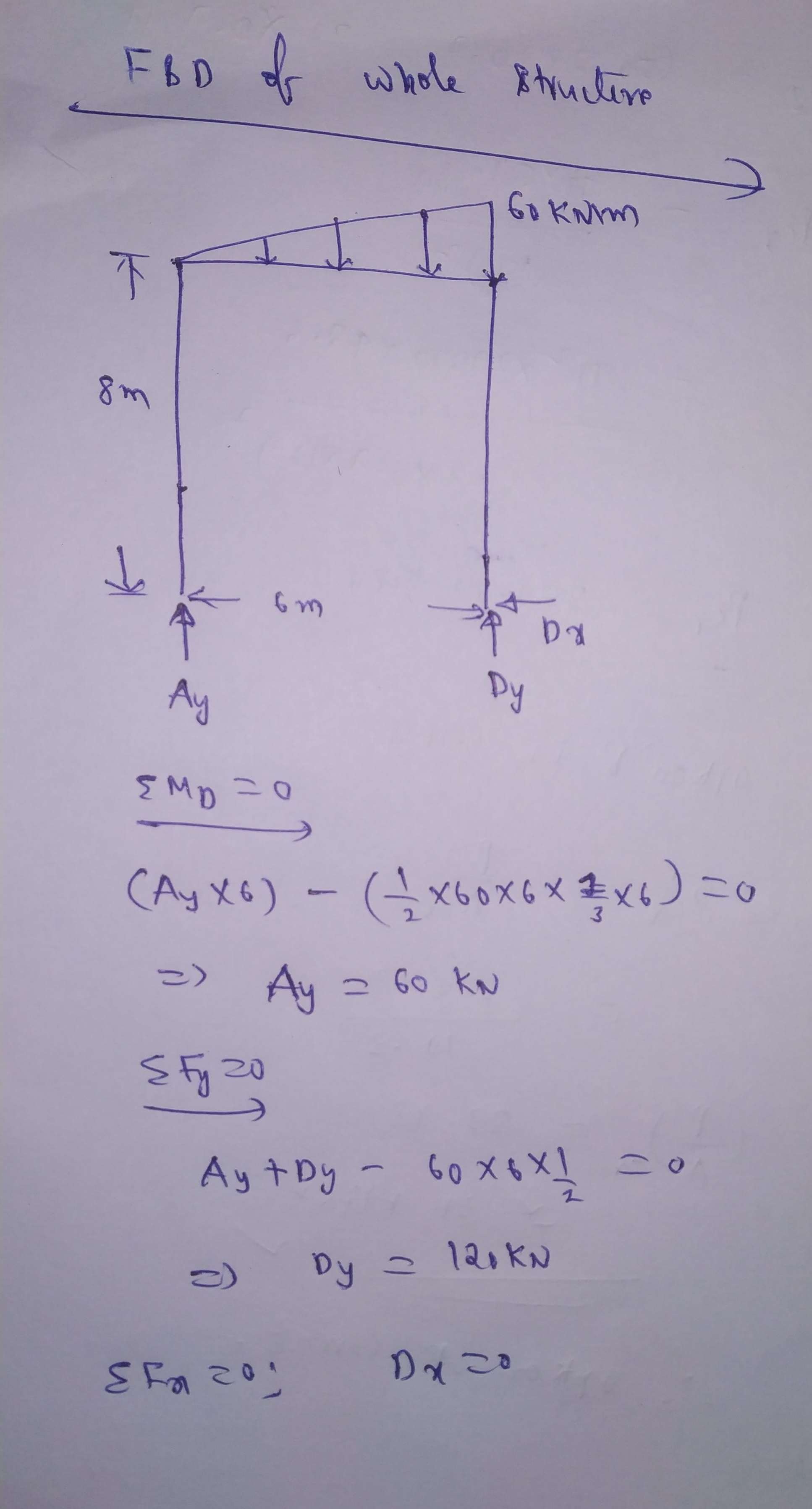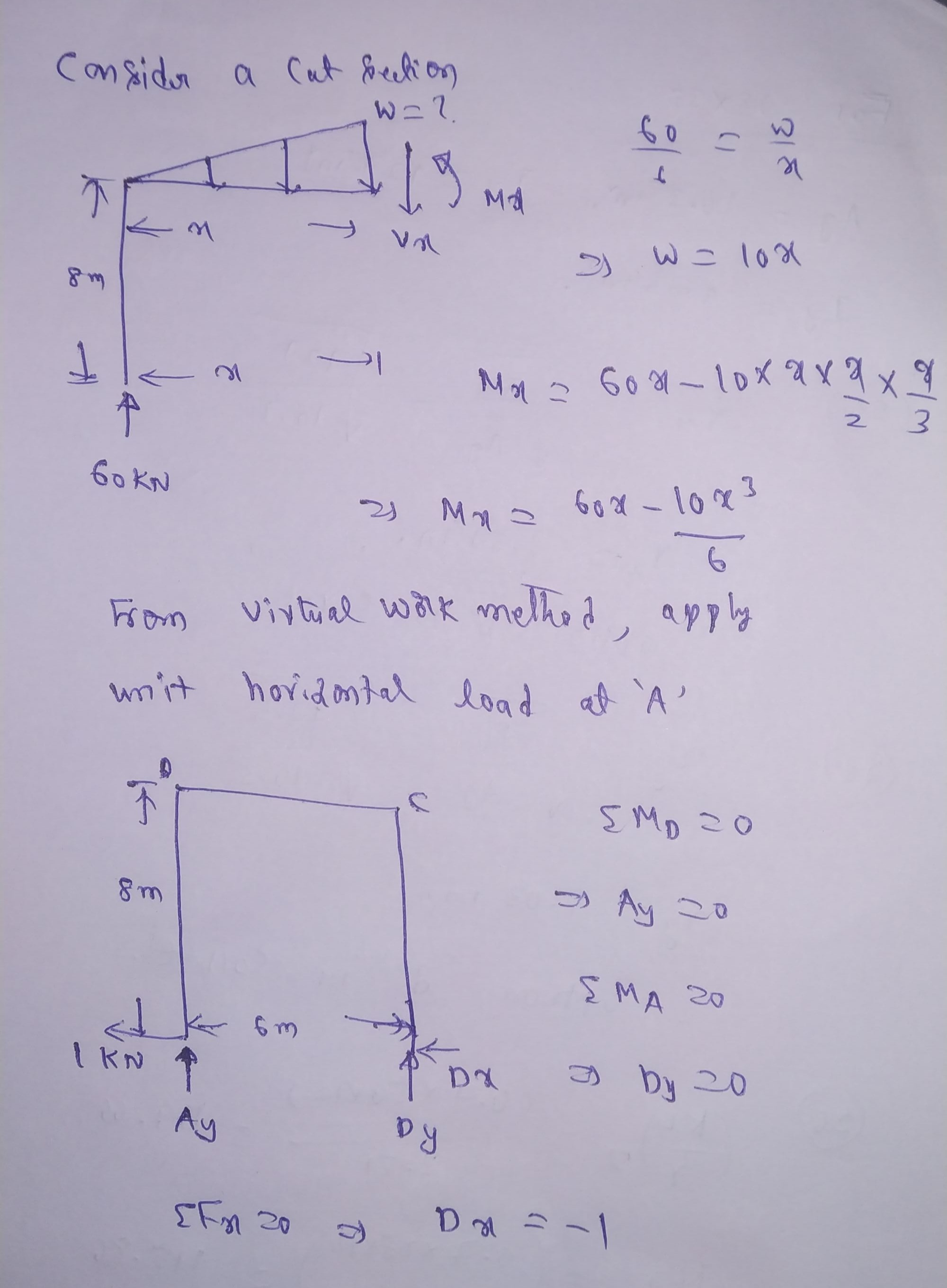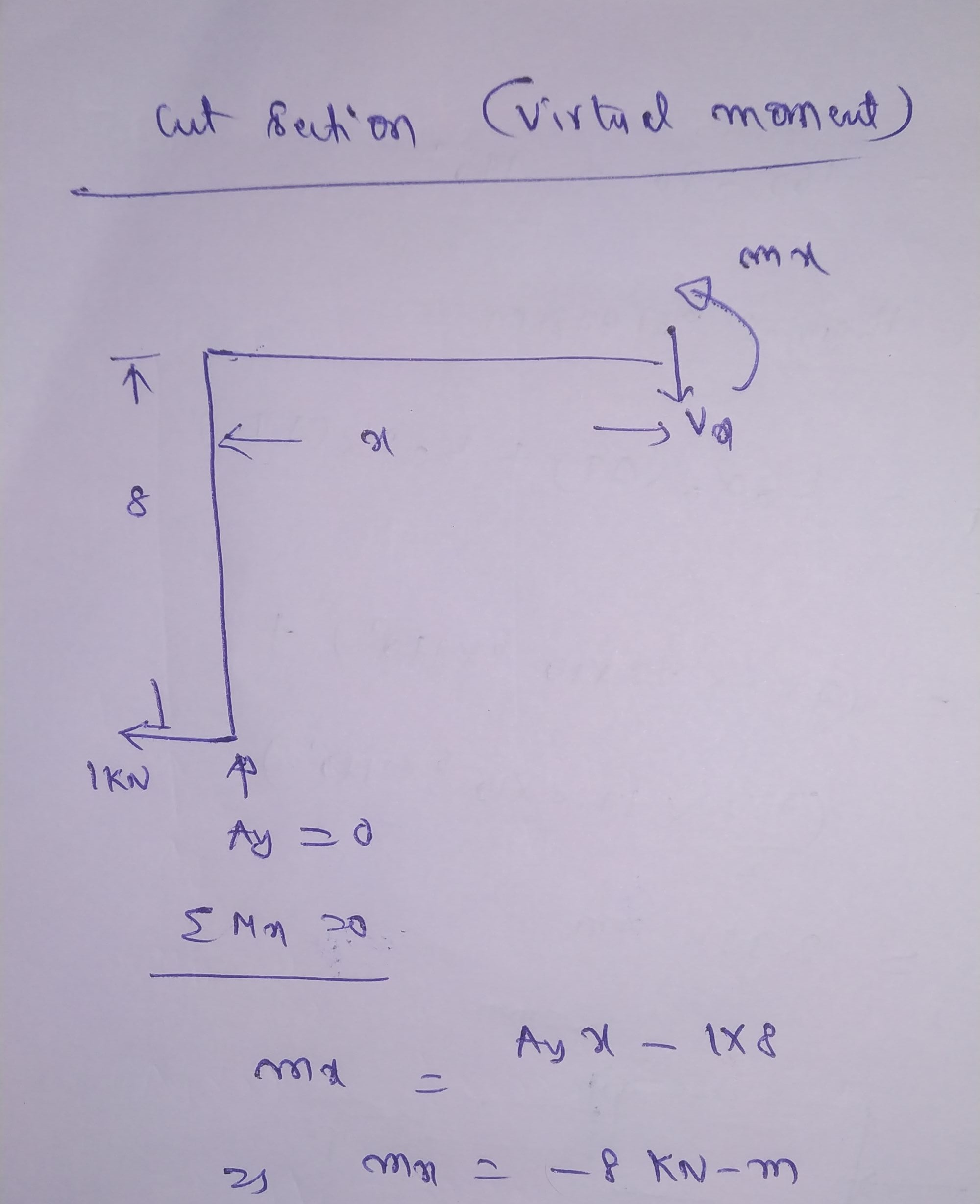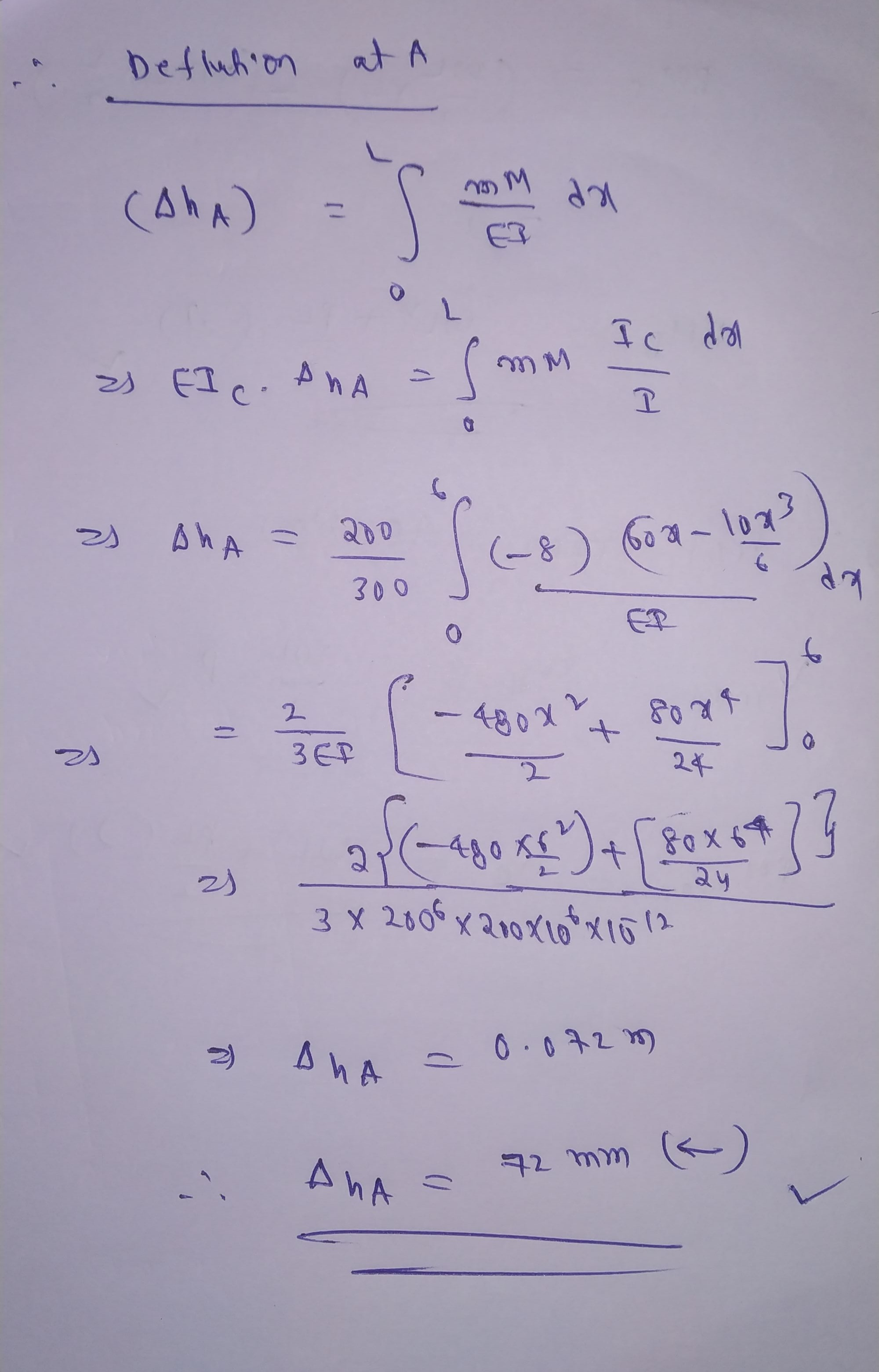#### Earn Coin

Coins can be redeemed for fabulous gifts.

Similar Homework Help Questions
• ### Problem 8-26 in Structural anaylsis edition 10 Determine the displacement and slope at point C. The...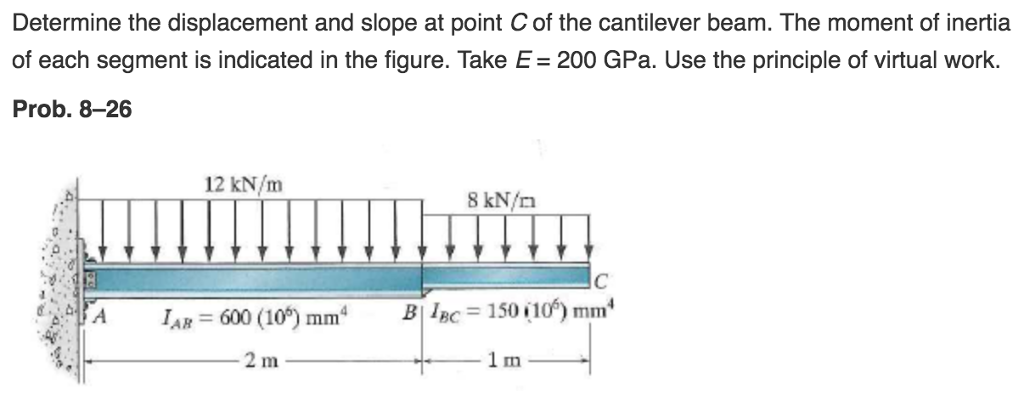Problem 8-26 in Structural anaylsis edition 10 Determine the displacement and slope at point C. The moment of inertia for each segment is indicated in the figure. Take E=200GPa. Use principle of virtual work. Determine the displacement and slope at point C of the cantilever beam. The moment of inertia of each segment is indicated in the figure. Take E 200 GPa. Use the principle of virtual work. Prob. 8-26 12 kN/m 8 kN/n - 600 (10) mm 150 i...

• ### Step by step solution Problem 3 Determine the displacement and slope at point C of the...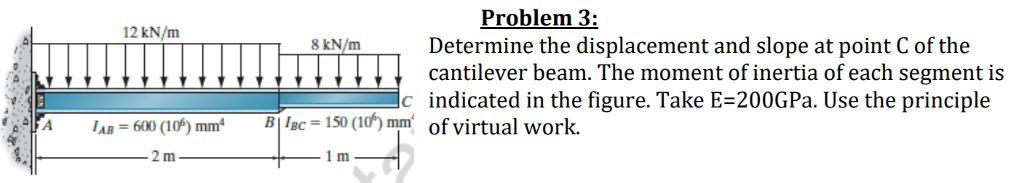Step by step solution Problem 3 Determine the displacement and slope at point C of the cantilever beam. The moment of inertia of each segment is 12 kN/m 8 kN/m c indicated in the figure. Take E-200GPa. Use the principle A AB 600 0) mmBBc1S0 (0) mm of virtual work. 1 m

• ### 8_57 please Probs. 8-55/56 -57. Determine the vertical displacement at A. Take E = 200 GPa,...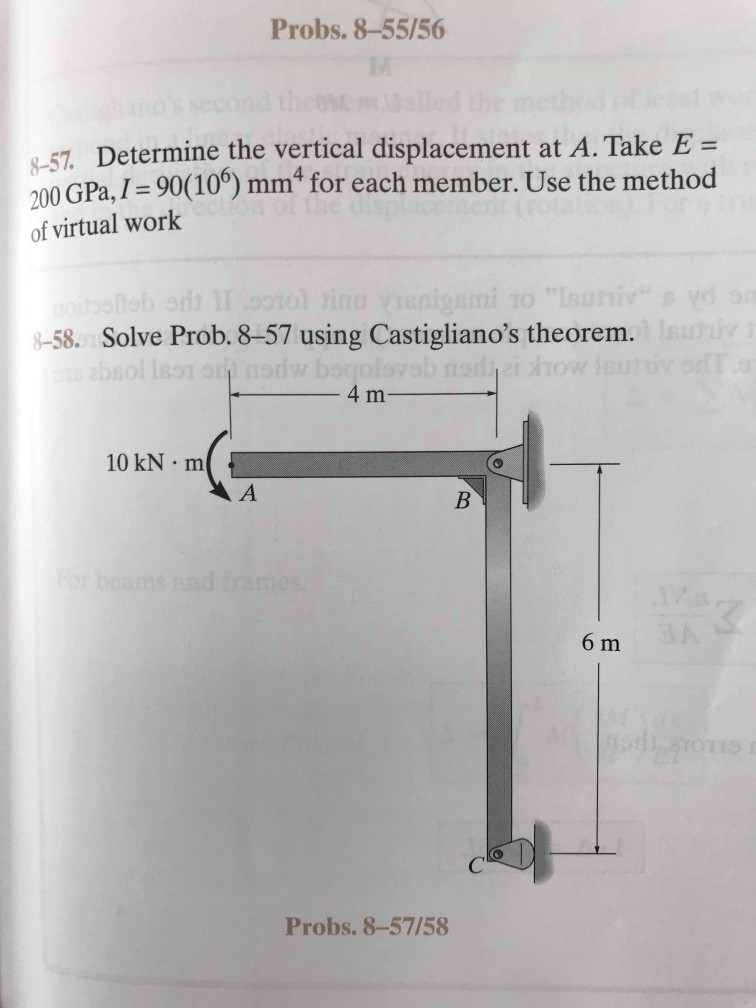8_57 please Probs. 8-55/56 -57. Determine the vertical displacement at A. Take E = 200 GPa, I = 90(10º) mmª for each member. Use the method of virtual work noitosftob orlt 1I 0010l tinu vIsnigemi to "Isuniv" 8-58. Solve Prob. 8–57 using Castigliano's theorem. boqolsv 4 m absol lssT or nody 10 kN · m For beams and frames 6 m Probs. 8-57/58

• ### Determine the slope at A. Take E = 29(10^3) The moment of inertia of each segmen...continues

Determine the slope at A. Take E = 29(10^3) The moment of inertia of each segment of the frame is indicated in the figure. Assume D is a pin support. Use the method ofvirtual work. From Structural Analysis Hibbeler 9-54

• ### 16-5. Determine the structure stiffness matrix K for the 200 GPa, are fixed. Take E and frame. Assume 1-300 105) mm,A 1...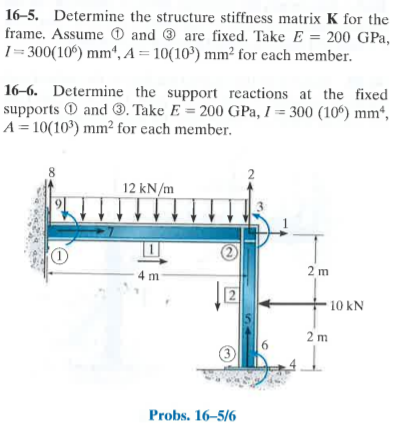16-5. Determine the structure stiffness matrix K for the 200 GPa, are fixed. Take E and frame. Assume 1-300 105) mm,A 10(10) mm2 for each member. 16-6. Determine the support reactions at the fixed supports D and . Take E-200 GPa,1 300 (10) mm, A 10(10) mm2 for each member. 12 kN/m 2 m 4 m 12 2 m Probs. 16-5/6 16-5. Determine the structure stiffness matrix K for the 200 GPa, are fixed. Take E and frame. Assume 1-300...

• ### Determine the slope at A. Take E = 29(10^3) The moment of inertia of each segment...continues

Determine the slope at A. Take E = 29(10^3) The moment of inertia of each segment of the frame is indicated in the figure. Assume D is a pin support. Use the method ofvirtual work. From Structural Analysis Hibbeler 9-54. Please don't just give me 9.55 which is solved using Castigliano's Theorem because I know how to do that one.

• ### 1. Determine the reactions at the supports. Assume the support at A is fixed and B...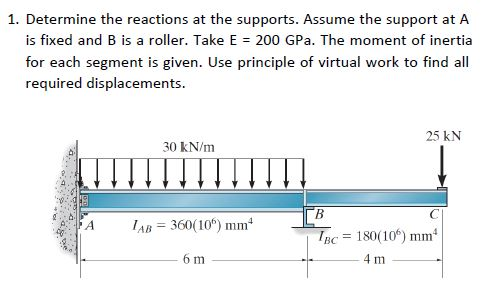1. Determine the reactions at the supports. Assume the support at A is fixed and B is a roller. Take E 200 GPa. The moment of inertia for each segment is given. Use principle of virtual work to find all required displacements. 25 kN 30 kN/m ГВ IAB 360(10) mm A TBC 180(10) mm 6 m 4 m

• ### Determine the moment inertia along the horizontal neutral axis for the cross section of the beam...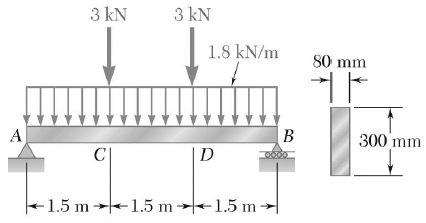Determine the moment inertia along the horizontal neutral axis for the cross section of the beam (in 106 mm4) and the maximum normal stress due to bending on a transverse section at C (in MPa) 3 KN 3 KN 1.8 kN/m 80 mm 11 A | В 300 mm D -1.5 m -1.5 m -1.5 m

• ### Portalio Problems-Virtal Work M ork Method Problem 3 For the frame structure below: a) Use the me...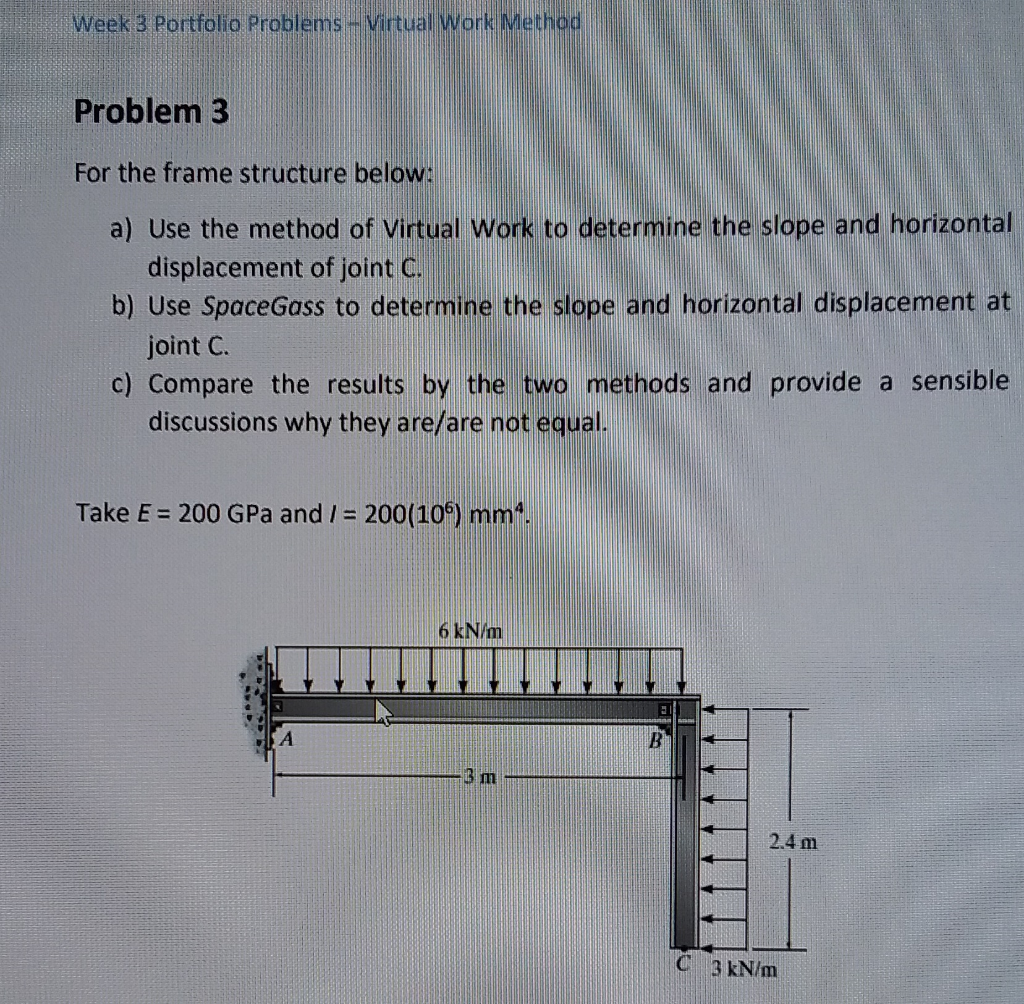Portalio Problems-Virtal Work M ork Method Problem 3 For the frame structure below: a) Use the method of Virtual Work to determine the slope and horizontal b) Use SpaceGass to determine the slope and horizontal displacement at c) Compare the results by the two methods and provide a sensible displacement of joint C joint C discussions why they are/are not equal. Take E 200 GPa and /- 200(10) mm 6 kN/m 3 m 2.4 m C 3 kN/m Portalio Problems-Virtal...

• ### Question 1 (25 points) Determine the horizontal displacement of point C. Assume the members are pin...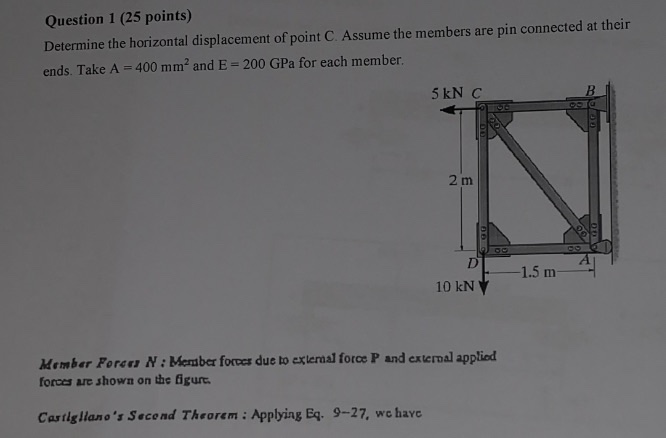Question 1 (25 points) Determine the horizontal displacement of point C. Assume the members are pin connected at their ends. Take A = 400 mm? and E = 200 GPa for each member. 5 kN C 2 m 1.5 m 10 kN Member Foron N: Member forces due to external force P and external applied forces are shown on the figurt. Castigliano's Second Theorem : Applying Eq. 9-27, we have